# GMAT Math : Calculating the length of the side of an equilateral triangle

## Example Questions

### Example Question #1 : Equilateral Triangles

If an equilateral triangle has a perimeter of, what is the length of each side?Cannot be determinedExplanation:

An equilateral triangle has three equal sides; therefore, to find the length of each side, divide the perimeter by: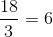### Example Question #2 : Equilateral Triangles

If the area of an equilateral is, given a height of, what is the base of the triangle?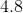Explanation:

We derive the equation of base of a triangle from the area of a triangle formula: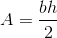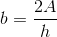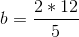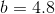### Example Question #3 : Equilateral Triangles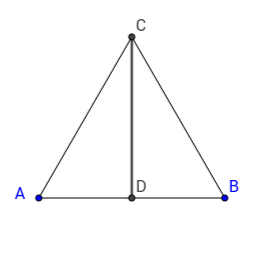The height of an equilateral triangle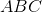is. What is the length of side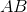?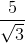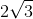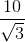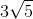Explanation:

Similarily, we can use the same formula for the height to find the length of the side of an equilateral triangle, which is given by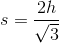, whereis the length of the height.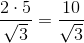### Example Question #4 : Equilateral Triangles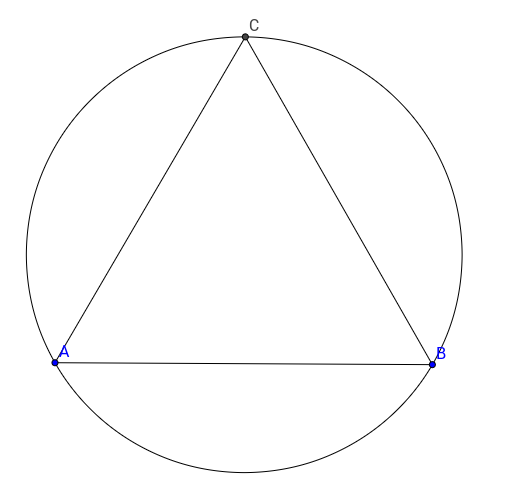Equilateral triangleis inscribed in a circle with radius, what is the length of a side of the triangle?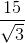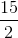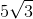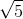Explanation:

Since we are given the radius, we should be able to find the height of the equilateral triangle. Indeed, the center of the circle is at the intersections of the heights of the triangle, and is locatedaway from the edge of a given height.

Therefore 5, the radius of the circle isof the height.

Therefore, the height must be.

From here, we can use the formula for the height of the equilateral triangle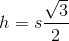, whereis the length of the height andis the length of a side of the equilateral triangle.

Therefore,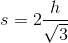, thenis the final answer.

Tired of practice problems?

Try live online GMAT prep today.